# R: Intro to ggplot()

ggplot() is a powerful graphing tool in R. While it is more complex to use than qplot(), its added complexity comes with advantages.

Don’t worry about the complexity, we are going to step into it slowly.

Let’s start by getting a data set. I am going to choose airquality from the R data sets package. You can find a list of data sets from that package here: Link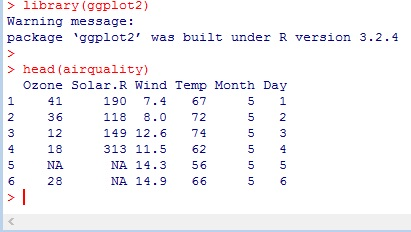We are going to manipulate some of this data, so first let us set the dataframe to a new variable.

Next, take a look at the structure – str() – we have 6 variables and 153 rows. The first thing I notice is that Month is an int – I don’t want that. I would rather have Month be a factor      (a categorical variable)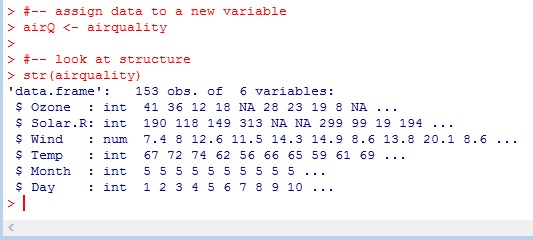Use factor() to set Month to a factor. Now look at str() readout again. There are 5 levels (months) represented in our data set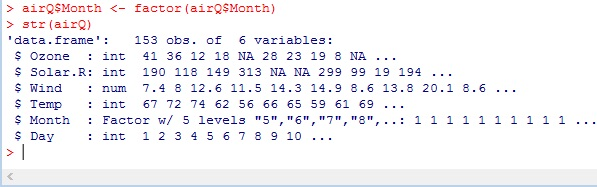## ggplot()

here is the basic syntax – ggplot(data, aes(x value, y value))+geom_[type of plot]

`ggplot(data = airQ,  aes(x = Wind, y = Temp)) + geom_point()`Now let’s add some color to the chart. Inside aes() – which stands for aesthetics – we are going to add color = Month

` ggplot(data = airQ, aes(x = Wind, y = Temp, color = Month)) + geom_point()`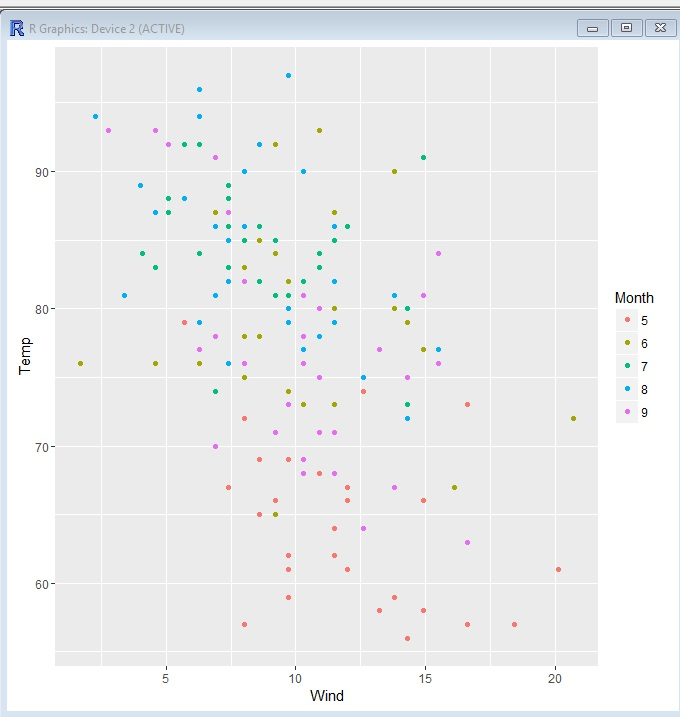`ggplot(data = airQ, aes(x = Wind, y = Temp, color = Month, size = Ozone)) + geom_point()`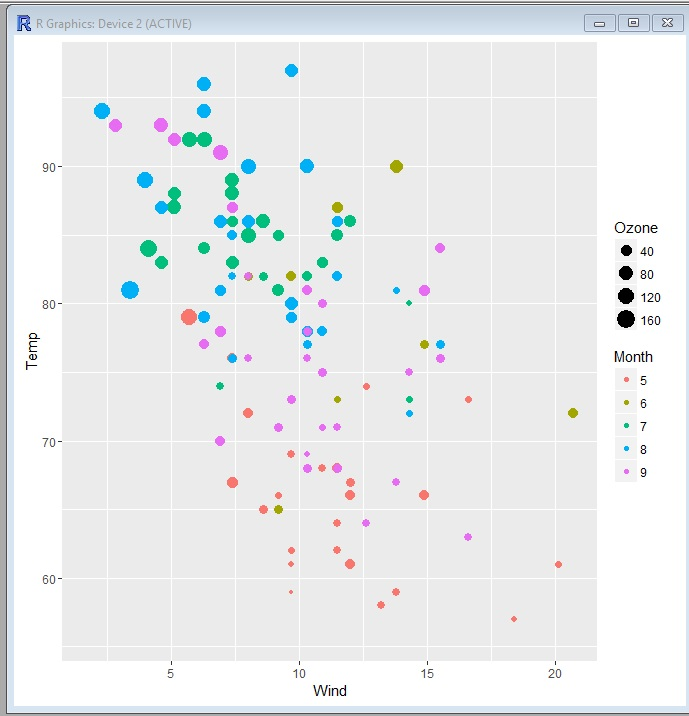## The Code

```library(ggplot2)

#-- assign data to a new variable
airQ <- airquality

#-- look at structure
str(airquality)

#-- set month as a factor
airQ\$Month <- factor(airQ\$Month)
str(airQ)

#-- plot wind vs temp - scatter plot
ggplot(data = airQ, aes(x = Wind, y = Temp)) + geom_point()

#-- plot add factor (Month) as a color
ggplot(data = airQ, aes(x = Wind, y = Temp, color = Month)) + geom_point()

#-- set Ozone as a size element
ggplot(data = airQ, aes(x = Wind, y = Temp, color = Month, size = Ozone)) + geom_point()```